×

Search anything:

# Check if given point is inside a convex polygon

#### Algorithms computational geometryIn this post, we discuss how to check if a given point is inside a convex polygon using the Graham scan algorithm and list application areas for the solution.

1. Problem Statement
2. Graham scan algorithm
3. Approach to solve the problem
4. Time and Space Complexity Analysis
5. Applications

Prerequisite: Graham scan algorithm

## Problem Statement

Problem statement: Given coordinates of n points of a convex polygon. Return true if the point (x, y) lies inside the polygon, false otherwise.

Input: Coordinates representing points on a polygon.
Output: True if the points lie inside the polygon, false otherwise.

#### Example.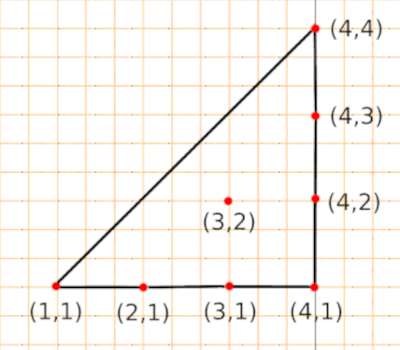Input: Points: {(1, 1), (2, 1), (3, 1), (4, 1), (4, 2), (4, 3), (4, 4)}, Query: (3, 2)
Output: True

Input: Points: {(1, 1), (2, 1), (3, 1), (4, 1), (4, 2), (4, 3), (4, 4)}, Query: (9, 2)
Output: False

To solve this problem we shall use Graham Scan Algorithm which is an efficient algorithm for finding a convex hull of a finite set of points.
Before we dive into the approach, we shall go through various concepts upon which this algorithm is based on to get a firmer grip.

Convex vs concave

A polygon is said to be convex if all its internal angles are less than ${180}^{\circ }$ and concave if they are greater than ${180}^{\circ }$.
We can also define a convex polygon as a simple polygon (without self intersections) such that any line segment between two points inside the polygon lies completely inside of it.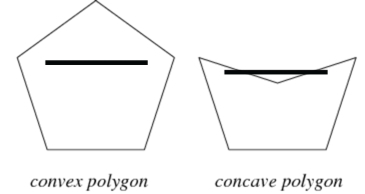Orientation.

We can determine if a tuple of 3 forms a clockwise, counter-clockwise turn or if they are collinear.

One way is by finding a determinant of the matrix formed by the three points. If it is positive then a->b->c is counter clockwise, otherwise if it is negative then it is clockwise, if it is zero then they are collinear.Polar angle.

The polar angle theta is the counter-clockwise angle from the x-axis on which a point xy-plane lies.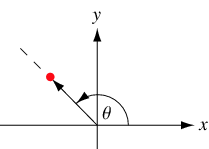## Graham scan algorithm

1. Given a set of points on a plane, we are to find a point with the lowest Y coordinate value, if they are more than one we select one with lower x coordinate value. We call it anchor point.
2. Sort all points based on the polar angle made by the anchor point. If two points make same anchor point, we sort using their distance from P.
3. Initialize a convex hull array with anchor point and the first element in the sorted array.
4. Iterate over each point in sorted array and see if traversing to a point from the previous makes a clockwise or counter-clockwise direction. If clockwise we reject it and move to the next. We repeat till we finish the sorted array.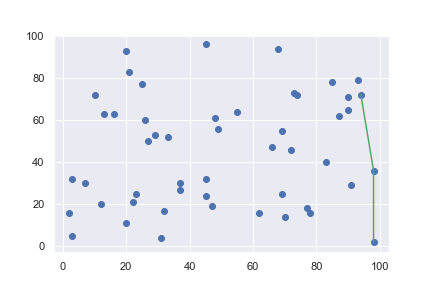## Approach to solve the problem

We use graham scan algorithm to obtain a convex hull.
If a points (x, y) lie inside the polygon, it wont be in the convex hull and therefore won't be in the newly generated set of points of the convex hull.
If points (x, y) lie outside the polygon, then they will be in the convex hull formed hence present in the set of points generated by the convex hull.

Note: A polygon consists of more than two line segments ordered in clockwise or anticlockwise manner. If a point lies on the left or right of the edges of a polygon whose edges are clockwise or anticlockwise we say that the point is inside the polygon.

Convex hull is the smallest convex set that encloses a given set of points.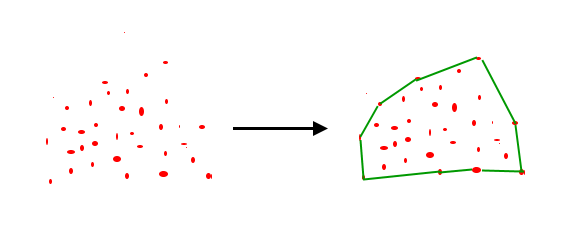### Steps

1. Sort the points in increasing order of their abscissa values and if the abscissa values of any two points are equal we sort them using their ordinate number.
2. Set bottom left point as start and top right point as end of convex hull.
3. Iterate over all points and find out points forming the convex polygon that lie between start and end points in the counterclockwise direction, store the points in a vector.
4. Check if query point exists in the vector, if so then it lies outside the convex hull, we return false.
5. If the point is not in the vector, then the point lies inside the convex hull so we return true.

### Code

``````#include<iostream>
#include<vector>
#include<algorithm>

using std::vector;
using std::pair;
using std::cout;
using std::endl;

class ConvexPoint{
private:
//check clockwise orientation
int cw(pair<int, int>& a, pair<int, int>& b, pair<int, int>& c){
int p = a.first * (b.second - c.second) + b.first * (c.second - a.second) + c.first * (a.second - b.second);
return p < 0ll;
}
//check counterclockwise orientation
int ccw(pair<int, int>& a, pair<int, int>& b, pair<int, int>& c){
int p = a.first * (b.second - c.second) + b.first * (c.second - a.second) + c.first * (a.second - b.second);
return p > 0ll;
}

//graham scan algorithm
vector<pair<int, int>> convexHull(vector<pair<int, int>>& v){
sort(v.begin(), v.end());

int n = v.size();
//if there are less than 3 edges, not valid
if(n <= 3)
return v;

//start and end points
pair<int, int> start = v;
pair<int, int> end = v[n-1];

vector<pair<int, int>> up, down;
up.push_back(start);
down.push_back(start);

for(int i = 1; i < n; i++){
if(i == n - 1 || !ccw(start, v[i], end)){
while(up.size() > 1 && ccw(up[up.size() - 2], up[up.size() - 1], v[i]))
up.pop_back();
up.push_back(v[i]);
}
if(i == n - 1 || !cw(start, v[i], end)){
while(down.size() > 1 && cw(down[down.size() - 2], down[down.size() - 1], v[i]))
down.pop_back();
down.push_back(v[i]);
}
}

//combine upper and lower half
for(int i = down.size() - 2; i > 0; i--)
up.push_back(down[i]);

up.resize(unique(up.begin(), up.end()) - up.begin());

//return convex hull points
return up;
}
public:
//check if point is in convex polygon
bool isInside(vector<pair<int, int>> points, pair<int, int> query){
//include query in polygon points
points.push_back(query);

//create a convex hull
points = convexHull(points);

//iterate over points
for(auto x : points){
//if query is in covex hull
if(x == query)
return false; //not in convex polygon
}
//inside polygon
return true;
}
};

int main(){
ConvexPoint cp;
vector<pair<int, int>> points = {{1, 1}, {2, 1}, {3, 1}, {4, 1}, {4, 2}, {4, 3}, {4, 4}};
//query
pair<int, int> q1 = {3, 2};
pair<int, int> q2 = {9, 2};

cout << cp.isInside(points, q1) << endl;
cout << cp.isInside(points, q2) << endl;
return 0;
}
``````

### Output.

``````1
0
``````

## Time and Space Complexity Analysis

This algorithm takes O(nlogn) time complexity in the worst case.

The space complexity is proportional to the number of n points to be stored, therefore it is O(n).

### Applications

• Polygon filling in raster devices.
• Hatching in drafting software
• Geospatial analysis software.
• Automated intruder detection systems.
• Fleet management systems.

With this article at OpenGenus, you must have the complete idea of how to Check if given point is inside a convex polygon.

#### Erick Lumunge

Erick is a passionate programmer with a computer science background who loves to learn about and use code to impact lives positively.

Check if given point is inside a convex polygon